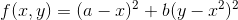623

35

155

30

# Optim.jl

Univariate and multivariate optimization in Julia.

Optim.jl is part of the JuliaNLSolvers family.

# Optimization

Optim.jl is a package for univariate and multivariate optimization of functions. A typical example of the usage of Optim.jl is

``````using Optim
rosenbrock(x) =  (1.0 - x)^2 + 100.0 * (x - x^2)^2
result = optimize(rosenbrock, zeros(2), BFGS())
``````

This minimizes the Rosenbrock functionwith a = 1, b = 100 and the initial values x=0, y=0. The minimum is at (a,a^2).

The above code gives the output

``````
* Status: success

* Candidate solution
Minimizer: [1.00e+00, 1.00e+00]
Minimum:   5.471433e-17

* Found with
Algorithm:     BFGS
Initial Point: [0.00e+00, 0.00e+00]

* Convergence measures
|x - x'|               = 3.47e-07 ≰ 0.0e+00
|x - x'|/|x'|          = 3.47e-07 ≰ 0.0e+00
|f(x) - f(x')|         = 6.59e-14 ≰ 0.0e+00
|f(x) - f(x')|/|f(x')| = 1.20e+03 ≰ 0.0e+00
|g(x)|                 = 2.33e-09 ≤ 1.0e-08

* Work counters
Seconds run:   0  (vs limit Inf)
Iterations:    16
f(x) calls:    53
∇f(x) calls:   53
``````

To get information on the keywords used to construct method instances, use the Julia REPL help prompt (`?`)

``````help?> LBFGS
search: LBFGS

LBFGS
≡≡≡≡≡≡≡

Constructor
=============

LBFGS(; m::Integer = 10,
alphaguess = LineSearches.InitialStatic(),
linesearch = LineSearches.HagerZhang(),
P=nothing,
precondprep = (P, x) -> nothing,
manifold = Flat(),
scaleinvH0::Bool = true && (typeof(P) <: Nothing))

LBFGS has two special keywords; the memory length m, and
the scaleinvH0 flag. The memory length determines how many
previous Hessian approximations to store. When scaleinvH0
== true, then the initial guess in the two-loop recursion
to approximate the inverse Hessian is the scaled identity,
as can be found in Nocedal and Wright (2nd edition) (sec.
7.2).

In addition, LBFGS supports preconditioning via the P and
precondprep keywords.

Description
=============

The LBFGS method implements the limited-memory BFGS
algorithm as described in Nocedal and Wright (sec. 7.2,
2006) and original paper by Liu & Nocedal (1989). It is a
quasi-Newton method that updates an approximation to the
Hessian using past approximations as well as the gradient.

References
============

•    Wright, S. J. and J. Nocedal (2006), Numerical
optimization, 2nd edition. Springer

•    Liu, D. C. and Nocedal, J. (1989). "On the
Limited Memory Method for Large Scale
Optimization". Mathematical Programming B. 45
(3): 503–528
``````

# Documentation

For more details and options, see the documentation

• STABLE — most recently tagged version of the documentation.
• LATEST — in-development version of the documentation.

# Installation

The package is a registered package, and can be installed with `Pkg.add`.

``````julia> using Pkg; Pkg.add("Optim")
``````

or through the `pkg` REPL mode by typing

``````] add Optim
``````

# Citation

If you use `Optim.jl` in your work, please cite the following.

``````@article{mogensen2018optim,
author  = {Mogensen, Patrick Kofod and Riseth, Asbj{\o}rn Nilsen},
title   = {Optim: A mathematical optimization package for {Julia}},
journal = {Journal of Open Source Software},
year    = {2018},
volume  = {3},
number  = {24},
pages   = {615},
doi     = {10.21105/joss.00615}
}
``````

04/04/2012